7780831891 [email protected]

Instructions: This Monk test contains JK Bank Height and Distance Problems WITH ANSWERS. This will help you in JK bank exam preparation.

This is a FREE online JK Bank Monk Test on topic JK Bank Height and Distance Problems with answer. You DO NOT pay money to anyone to attend this test.
Each question carries 1 mark, no negative marks.
DO NOT refresh the page.
All the best :-).

### #1. Two ships are sailing in the sea on the two sides of a lighthouse. The angle of elevation of the top of the lighthouse is observed from the ships are 30° and 45° respectively. If the lighthouse is 100 m high, the distance between the two ships is:

Explanation:

Let AB be the lighthouse and C and D be the positions of the ships.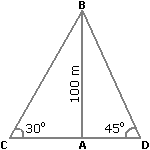Then, AB = 100 m,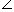ACB = 30° andADB = 45°.

 AB = tan 30° = 1AC = AB x √3 = 100√3 m. AC √3
 AB = tan 45° = 1AD = AB = 100 m. ADCD = (AC AD) = (100√3  100) m = 100(√3  1) = (100 x 2.73) m = 273 m.

### #2. A man standing at a point P is watching the top of a tower, which makes an angle of elevation of 30° with the man's eye. The man walks some distance towards the tower to watch its top and the angle of the elevation becomes 60°. What is the distance between the base of the tower and the point P?

Explanation:

One of AB, AD and CD must have given.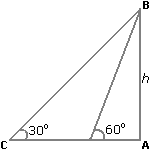### #3. The angle of elevation of a ladder leaning against a wall is 60° and the foot of the ladder is 4.6 m away from the wall. The length of the ladder is:

Explanation:

Let AB be the wall and BC be the ladder.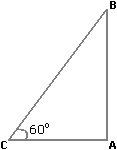Then,ACB = 60° and AC = 4.6 m.

 AC = cos 60° = 1 BC 2BC = 2 x AC = (2 x 4.6) m = 9.2 m.

### #4. An observer 1.6 m tall is 203 away from a tower. The angle of elevation from his eye to the top of the tower is 30°. The height of the tower is:

Explanation:

Let AB be the observer and CD be the tower.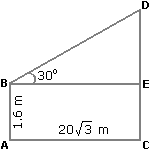Draw BE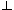CD.

Then, CE = AB = 1.6 m,

BE = AC = 203 m.

 DE = tan 30° = 1 BE 3DE = 203 m = 20 m. 3CD = CE DE = (1.6 20) m = 21.6 m.

### #5. From a point P on a level ground, the angle of elevation of the top tower is 30°. If the tower is 100 m high, the distance of point P from the foot of the tower is:

Explanation:

Let AB be the tower.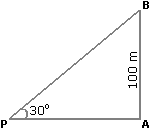Then,APB = 30° and AB = 100 m.

 AB = tan 30° = 1 AP 3AP = (AB x 3) m = 1003 m = (100 x 1.73) m = 173 m.
error decoding

### #6. The angle of elevation of the sun, when the length of the shadow of a tree 3 times the height of the tree, is: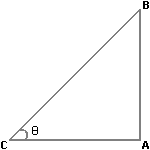LetACB =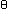.
 Then, AC = √3cot= √3 AB= 30°.